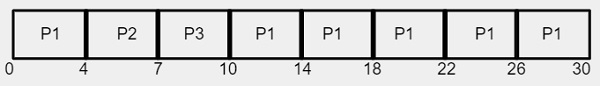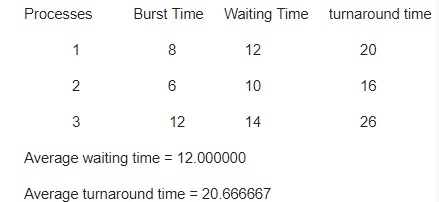# C Program for Round Robin scheduling

We are given with the n processes with their corresponding burst time and time quantum and the task is to find the average waiting time and average turnaround time and display the result.

What is Round Robin Scheduling?

Round robin is a CPU scheduling algorithm that is designed especially for time sharing systems. It is more like a FCFS scheduling algorithm with one change that in Round Robin processes are bounded with a quantum time size. A small unit of time is known as Time Quantum or Time Slice. Time quantum can range from 10 to 100 milliseconds. CPU treat ready queue as a circular queue for executing the processes with given time slice. It follows preemptive approach because fixed time are allocated to processes. The only disadvantage of it is overhead of context switching.

What we need to calculate?

Completion Time is the time required by the process to complete its execution

Turnaround Time is the time interval between the submission of a process and its completion.

Turnaround Time = completion of a process – submission of a process

Waiting Time is the difference between turnaround time and burst time

Waiting Time = turnaround time – burst time

## Example

We are given with 3 processes P1, P2 and P3 with their corresponding burst time as 24, 3 and 3

ProcessBurst Time
P124
P23
P33

Since the time quantum is of 4 milliseconds, process P1 gets the first 4 milliseconds but it requires another 20 millisecond to complete its execution but CPU will preempt it after the first time quantum and CPU will be allocated to the next process P2. As shown in the table, Process P2 requires only 3 milliseconds to complete its execution so CPU will be allocated for time quantum of 3 milliseconds only instead of 4 milliseconds.Using the Gantt chart, Average waiting time is calculated as given below −

Average waiting time = 17/3 = 5.66 milliseconds

## Algorithm

Start
Step 1-> In function int turnarroundtime(int processes[], int n, int bt[], int wt[], int tat[])
Loop For i = 0 and i < n and i++
Set tat[i] = bt[i] + wt[i]
return 1
Step 2-> In function int waitingtime(int processes[], int n, int bt[], int wt[], int quantum)
Declare rem_bt[n]
Loop For i = 0 and i < n and i++
Set rem_bt[i] = bt[i]
Set t = 0
Loop While (1)
Set done = true
Loop For i = 0 and i < n and i++
If rem_bt[i] > 0 then,
Set done = false
If rem_bt[i] > quantum then,
Set t = t + quantum
Set rem_bt[i] = rem_bt[i] - quantum
Else
Set t = t + rem_bt[i]
Set wt[i] = t - bt[i]
Set rem_bt[i] = 0
If done == true then,
Break
Step 3->In function int findavgTime(int processes[], int n, int bt[], int quantum)
Declare and initialize wt[n], tat[n], total_wt = 0, total_tat = 0
Call function waitingtime(processes, n, bt, wt, quantum)
Call function turnarroundtime(processes, n, bt, wt, tat)
Print "Processes Burst Time Waiting Time turnaround time "
Loop For i=0 and i<n and i++
Set total_wt = total_wt + wt[i]
Set total_tat = total_tat + tat[i]
Print the value i+1, bt[i], wt[i], tat[i]
Print "Average waiting time = total_wt / n
Print "Average turnaround time =total_tat / n
Step 4-> In function int main()
Delcare and initialize processes[] = { 1, 2, 3}
Declare and initialize n = sizeof processes / sizeof processes
Declare and initialize burst_time[] = {8, 6, 12}
Set quantum = 2
Call function findavgTime(processes, n, burst_time, quantum)

## Example

Live Demo

#include <stdio.h>
// Function to calculate turn around time
int turnarroundtime(int processes[], int n,
int bt[], int wt[], int tat[]) {
// calculating turnaround time by adding
// bt[i] + wt[i]
for (int i = 0; i < n ; i++)
tat[i] = bt[i] + wt[i];
return 1;
}
// Function to find the waiting time for all
// processes
int waitingtime(int processes[], int n,
int bt[], int wt[], int quantum) {
// Make a copy of burst times bt[] to store remaining
// burst times.
int rem_bt[n];
for (int i = 0 ; i < n ; i++)
rem_bt[i] = bt[i];
int t = 0; // Current time
// Keep traversing processes in round robin manner
// until all of them are not done.
while (1) {
bool done = true;
// Traverse all processes one by one repeatedly
for (int i = 0 ; i < n; i++) {
// If burst time of a process is greater than 0
// then only need to process further
if (rem_bt[i] > 0) {
done = false; // There is a pending process
if (rem_bt[i] > quantum) {
// Increase the value of t i.e. shows
// how much time a process has been processed
t += quantum;
// Decrease the burst_time of current process
// by quantum
rem_bt[i] -= quantum;
}
// If burst time is smaller than or equal to
// quantum. Last cycle for this process
else {
// Increase the value of t i.e. shows
// how much time a process has been processed
t = t + rem_bt[i];
// Waiting time is current time minus time
// used by this process
wt[i] = t - bt[i];
// As the process gets fully executed
// make its remaining burst time = 0
rem_bt[i] = 0;
}
}
}
// If all processes are done
if (done == true)
break;
}
return 1;
}
// Function to calculate average time
int findavgTime(int processes[], int n, int bt[],
int quantum) {
int wt[n], tat[n], total_wt = 0, total_tat = 0;
// Function to find waiting time of all processes
waitingtime(processes, n, bt, wt, quantum);
// Function to find turn around time for all processes
turnarroundtime(processes, n, bt, wt, tat);
// Display processes along with all details
printf("Processes Burst Time Waiting Time turnaround time");
// Calculate total waiting time and total turn
// around time
for (int i=0; i<n; i++) {
total_wt = total_wt + wt[i];
total_tat = total_tat + tat[i];
printf("\t%d\t\t\t%d\t\t\t%d\t\t\t%d",i+1, bt[i], wt[i], tat[i]);
}
printf("Average waiting time = %f", (float)total_wt / (float)n);
printf("Average turnaround time = %f", (float)total_tat / (float)n);
return 1;
}
// main function
int main() {
// process id's
int processes[] = { 1, 2, 3};
int n = sizeof processes / sizeof processes;
// Burst time of all processes
int burst_time[] = {8, 6, 12};
// Time quantum
int quantum = 2;
findavgTime(processes, n, burst_time, quantum);
return 0;
}

## Output